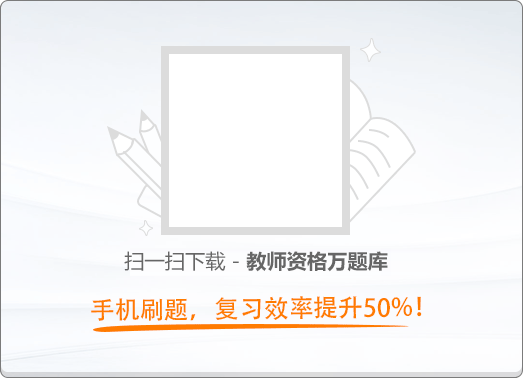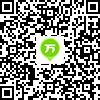120:002017年11月《中学综合素质》真题

1
(单项选择题)

• A.

教学理念偏失

• B.

教学态度不端

• C.

教学评价不当

• D.

教育行为失落

• A
• B
• C
• D

2
(单项选择题)

• A.

尊重了学生的学习要求

• B.

培养了学生的探究意识

• C.

激发了学生的学习兴趣

• D.

纠正了学生的研究方法

• A
• B
• C
• D

3
(单项选择题)

• A.

“你读得很响亮，若再有感情一点就好了，你再试试。”

• B.

“刚才这位同学概括的不准确，还是我来吧。”

• C.

“这位同学的发言并没有重复前面同学说过的话，有自己的观点，非常好！”

• D.

“请大家想一想，刚才这两位同学报告的结论，有何不同？”

• A
• B
• C
• D

4
(单项选择题)

• A.

忽视了学生的发展性

• B.

忽视了学生的差异性

• C.

尊重了学生的创造性

• D.

尊重了学生的自主性

• A
• B
• C
• D

5
(单项选择题)

《国家中长期教育改革和发展规划纲要（2010～2020年）》提出，要把教育摆在优先发展的战略地位。对于教育优先发展战略的理解，下列选项不恰当的是（　）。

• A.

财政资金优先保障教育投入

• B.

社会资源优先向教育领域倾斜

• C.

经济社会发展规划优先安排教育发展

• D.

公共资源优先满足教育和人力资源开发需要

• A
• B
• C
• D

6
(单项选择题)

• A.

合法，教师有权批评和管教学生

• B.

不合法，侵犯了邹某的财产权

• C.

合法，教师无权没收学生的手机

• D.

不合法，侵犯了邹某的隐私权

• A
• B
• C
• D

7
(单项选择题)

• A.

合法，有利于促进薄弱学校本身的内涵发展

• B.

合法，有利于实现优质教育资源的均衡共享

• C.

不合法，不得以任何方式改变或变相改变公办学校的性质

• D.

不合法，不得以任何方式或理由规避公开招标的原则要求

• A
• B
• C
• D

8
(单项选择题)

• A.

合法，有利于教师科学研究权的落实和保障

• B.

合法，有利于教师教学自由权的落实和保障

• C.

不合法，侵犯了其他教师享受平等待遇的权利

• D.

不合法，违反了教师考核评价的客观公正原则

• A
• B
• C
• D

9
(单项选择题)

• A.

学校和宋某

• B.

宋某

• C.

学校和李某的监护人

• D.

李某的监护人和韩某的监护人

• A
• B
• C
• D

10
(单项选择题)

• A.

撤销林某的监护人资格

• B.

给予林某行政处分

• C.

责令林某赔礼道歉

• D.

要求林某赔偿损失

• A
• B
• C
• D

11
(单项选择题)

• A.

聘任优秀的教师担任法制教育的兼职教师

• B.

聘任当地派出所干警担任校外法律辅导员

• C.

要求未成年人的父母配合开展法制教育

• D.

要求班主任承担对未成年人的监护责任

• A
• B
• C
• D

12
(单项选择题)

• A.

学校无法律责任

• B.

学校应承担过错责任

• C.

学校应承担无过错责任

• D.

学校应承担补充责任

• A
• B
• C
• D

13
(单项选择题)

• A.

偏爱张刚，未能关注其他同学

• B.

专心教学，不必承担其他责任

• C.

细心观察，适时捕捉教育契机

• D.

侵犯隐私，干扰学生私人生活

• A
• B
• C
• D

14
(单项选择题)

• A.

教师需要不断提高教育教学水平

• B.

教师发展首先应该考虑团结合作

• C.

中学教师不需要深厚的理论知识

• D.

中学教师应该合理安排闲暇时间

• A
• B
• C
• D

15
(单项选择题)

• A.

正确，有利于遏制其他同学的类似行为

• B.

应该维护教师的尊严和威信

• C.

不正确，不应该对此类学生如此宽容

• D.

不正确，不利于构建良好的师生关系

• A
• B
• C
• D

16
(单项选择题)

• A.

有利于促进家校合作

• B.

有利于履行班主任职责

• C.

影响了其他学生的成长

• D.

影响了校长的廉洁从教

• A
• B
• C
• D

17
(单项选择题)

• A.

百米赛跑世界纪录在10秒之内

• B.

通常一节课的时间是45分钟

• C.

从甲地步行到乙地需要2小时

• D.

新闻联播节目每天19时开始

• A
• B
• C
• D

18
(单项选择题)

• A.

培根

• B.

洪堡

• C.

魏格纳

• D.

达尔文

• A
• B
• C
• D

19
(单项选择题)

• A.

普法战争时期

• B.

英法百年战争时期

• C.

第一次世界大战时期

• D.

欧洲三十年战争时期

• A
• B
• C
• D

20
(单项选择题)

• A.

大使馆

• B.

领事馆

• C.

公使馆

• D.

代办处

• A
• B
• C
• D

21
(单项选择题)

• A.

天王星

• B.

海王星

• C.

土星

• D.

木星

• A
• B
• C
• D

22
(单项选择题)

• A.

《奥赛罗》

• B.

《雅典的泰门》

• C.

《麦克白》

• D.

《威尼斯商人》

• A
• B
• C
• D

23
(单项选择题)

《人间词话》是著名学者王国维的一部文学理论著作。他提出的关于创作原创和批评标准的核心理论是（　）。

• A.

“气质”说

• B.

“境界”说

• C.

“神韵”说

• D.

“格律”说

• A
• B
• C
• D

24
(单项选择题)

• A.

咏叹调是一种“朗诵”式的歌曲，依附于歌剧情节，相当于戏剧中的“对白”

• B.

咏叹调常安排在剧情发展的重要时刻，着重表现剧中人在特定情景中的情感

• C.

咏叹调旋律优美，故也常常单独在音乐会上演唱

• D.

咏叹调富有艺术魅力，要求较高驾驭声音的技巧

• A
• B
• C
• D

25
(单项选择题)• A.

A

• B.

B

• C.

C

• D.

D

• A
• B
• C
• D

26
(单项选择题)

• A.

“拼写和句法”错误

• B.

“句法和连接”错误

• C.

“拼写和语法”错误

• D.

“语法和连接”错误

• A
• B
• C
• D

27
(单项选择题)

• A.

大纲模式

• B.

幻灯片模式

• C.

幻灯片浏览模式

• D.

幻灯片放映模式

• A
• B
• C
• D

28
(单项选择题)

• A.

“军人”和“军医”

• B.

“杨树”和“柳树”

• C.

“蛋糕”和“面包”

• D.

“作家”和“画家”

• A
• B
• C
• D

29
(单项选择题)• A.

A

• B.

B

• C.

C

• D.

D

• A
• B
• C
• D